# morse tutorial

#### 2018-01-29

The package `morse` is devoted to the analysis of data from standard toxicity tests. It provides a simple workflow to explore/visualize a dataset, and compute estimations of risk assessment indicators. This document illustrates a typical use of `morse` on survival and reproduction data, which can be followed step-by-step to analyze new datasets.

# Survival data analysis at target time (TT)

The following example shows all the steps to perform survival analysis on standard toxicity test data and to produce estimated values of the \(LC_x\). We will use a dataset of the library named `cadmium2`, which contains both survival and reproduction data from a chronic laboratory toxicity test. In this experiment, snails were exposed to six concentrations of a metal contaminant (cadmium) during 56 days.

## Step 1: check the structure and the dataset integrity

The data from a survival toxicity test should be gathered in a `data.frame` with a specific layout. This is documented in the paragraph on `survData` in the reference manual, and you can also inspect one of the datasets provided in the package (e.g., `cadmium2`). First, we load the dataset and use the function `survDataCheck` to check that it has the expected layout:

``````data(cadmium2)
``## No message``

The output `## No message` just informs that the dataset is well-formed.

## Step 2: create a `survData` object

The class `survData` corresponds to survival data and is the basic layout used for the subsequent operations. Note that if the call to `survDataCheck` reports no error (i.e., `## No message`), it is guaranteed that `survData` will not fail.

``````dat <- survData(cadmium2)
``````## # A tibble: 6 x 6
##    conc  time Nsurv Nrepro replicate Ninit
##   <dbl> <int> <int>  <int>     <dbl> <int>
## 1     0     0     5      0         1     5
## 2     0     3     5    262         1     5
## 3     0     7     5    343         1     5
## 4     0    10     5    459         1     5
## 5     0    14     5    328         1     5
## 6     0    17     5    742         1     5``````

## Step 3: visualize your dataset

The function `plot` can be used to plot the number of surviving individuals as a function of time for all concentrations and replicates.

``plot(dat, pool.replicate = FALSE)``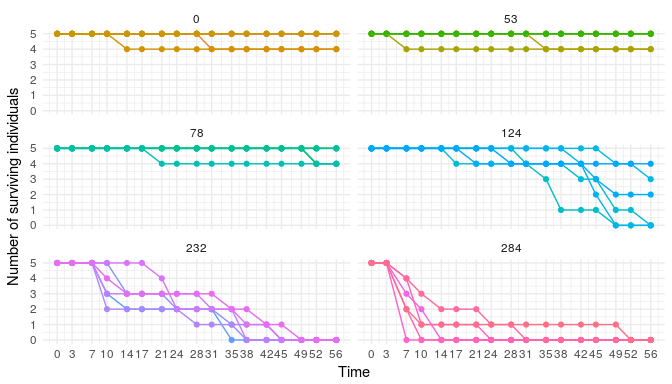Two graphical styles are available, `"generic"` for standard `R` plots or `"ggplot"` to call package `ggplot2` (default). If argument `pool.replicate` is `TRUE`, datapoints at a given time-point and a given concentration are pooled and only the mean number of survivors is plotted. To observe the full dataset, we set this option to `FALSE`.

By fixing the concentration at a (tested) value, we can visualize one subplot in particular:

``````plot(dat, concentration = 124, addlegend = TRUE,
pool.replicate = FALSE, style ="generic")``````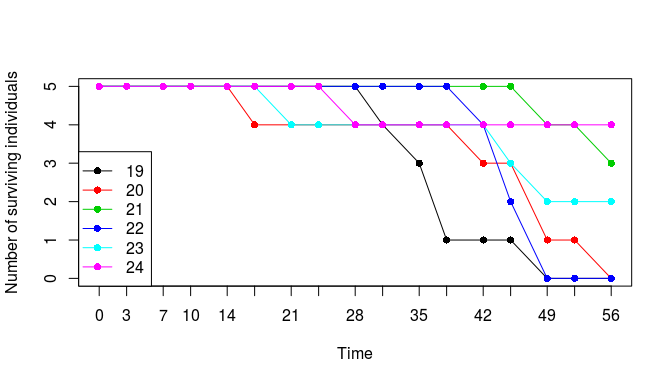We can also plot the survival rate, at a given time-point, as a function of concentration, with binomial confidence intervals around the data. This is achieved by using function `plotDoseResponse` and by fixing the option `target.time` (default is the end of the experiment).

``plotDoseResponse(dat, target.time = 21, addlegend = TRUE)``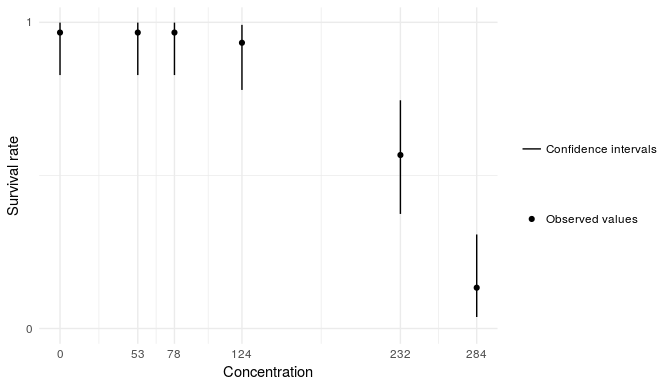Function `summary` provides some descriptive statistics on the experimental design.

``summary(dat)``
``````##
## Number of replicates per time and concentration:
##      time
## conc  0 3 7 10 14 17 21 24 28 31 35 38 42 45 49 52 56
##   0   6 6 6  6  6  6  6  6  6  6  6  6  6  6  6  6  6
##   53  6 6 6  6  6  6  6  6  6  6  6  6  6  6  6  6  6
##   78  6 6 6  6  6  6  6  6  6  6  6  6  6  6  6  6  6
##   124 6 6 6  6  6  6  6  6  6  6  6  6  6  6  6  6  6
##   232 6 6 6  6  6  6  6  6  6  6  6  6  6  6  6  6  6
##   284 6 6 6  6  6  6  6  6  6  6  6  6  6  6  6  6  6
##
## Number of survivors (sum of replicates) per time and concentration:
##      0  3  7 10 14 17 21 24 28 31 35 38 42 45 49 52 56
## 0   30 30 30 30 29 29 29 29 29 28 28 28 28 28 28 28 28
## 53  30 30 29 29 29 29 29 29 29 29 28 28 28 28 28 28 28
## 78  30 30 30 30 30 30 29 29 29 29 29 29 29 29 29 27 27
## 124 30 30 30 30 30 29 28 28 27 26 25 23 21 18 11 11  9
## 232 30 30 30 22 18 18 17 14 13 12  8  4  3  1  0  0  0
## 284 30 30 15  7  4  4  4  2  2  1  1  1  1  1  1  0  0``````

## Step 4: fit an exposure-response model to the survival data at target time

Now we are ready to fit a probabilistic model to the survival data, in order to describe the relationship between the concentration in chemical compound and survival rate at the target time. Our model assumes this latter is a log-logistic function of the former, from which the package delivers estimates of the parameters. Once we have estimated the parameters, we can then calculate the \(LC_x\) values for any \(x\). All this work is performed by the `survFitTT` function, which requires a `survData` object as input and the levels of \(LC_x\) we want:

``````fit <- survFitTT(dat,
target.time = 21,
lcx = c(10, 20, 30, 40, 50))``````

The returned value is an object of class `survFitTT` providing the estimated parameters as a posterior1 distribution, which quantifies the uncertainty on their true value. For the parameters of the models, as well as for the \(LC_x\) values, we report the median (as the point estimated value) and the 2.5 % and 97.5 % quantiles of the posterior (as a measure of uncertainty, a.k.a. credible intervals). They can be obtained by using the `summary` method:

``summary(fit)``
``````## Summary:
##
## The  loglogisticbinom_3  model with a binomial stochastic part was used !
##
## Priors on parameters (quantiles):
##
##         50%      2.5%     97.5%
## b 1.000e+00 1.259e-02 7.943e+01
## d 5.000e-01 2.500e-02 9.750e-01
## e 1.227e+02 5.390e+01 2.793e+02
##
## Posteriors of the parameters (quantiles):
##
##         50%      2.5%     97.5%
## b 8.580e+00 4.023e+00 1.625e+01
## d 9.570e-01 9.099e-01 9.862e-01
## e 2.364e+02 2.098e+02 2.533e+02
##
## Posteriors of the LCx (quantiles):
##
##            50%      2.5%     97.5%
## LC10 1.831e+02 1.267e+02 2.139e+02
## LC20 2.012e+02 1.538e+02 2.261e+02
## LC30 2.142e+02 1.747e+02 2.350e+02
## LC40 2.255e+02 1.928e+02 2.436e+02
## LC50 2.364e+02 2.098e+02 2.533e+02``````

If the inference went well, it is expected that the difference between quantiles in the posterior will be reduced compared to the prior, meaning that the data were helpful to reduce the uncertainty on the true value of the parameters. This simple check can be performed using the summary function.

The fit can also be plotted:

``plot(fit, log.scale = TRUE, adddata = TRUE,   addlegend = TRUE)``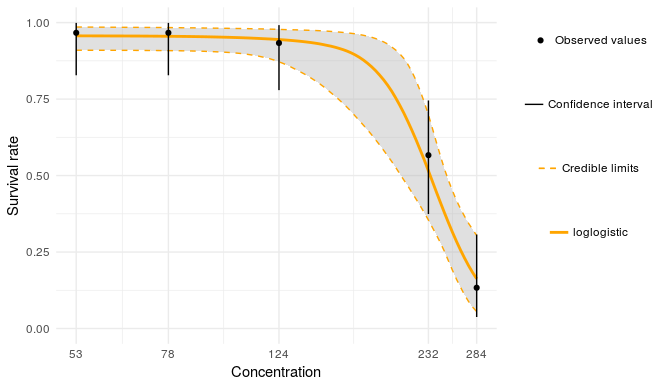This representation shows the estimated relationship between concentration of chemical compound and survival rate (orange curve). It is computed by choosing for each parameter the median value of its posterior. To assess the uncertainty on this estimation, we compute many such curves by sampling the parameters in the posterior distribution. This gives rise to the grey band, showing for any given concentration an interval (called credible interval) containing the survival rate 95% of the time in the posterior distribution. The experimental data points are represented in black and correspond to the observed survival rate when pooling all replicates. The black error bars correspond to a 95% confidence interval, which is another, more straightforward way to bound the most probable value of the survival rate for a tested concentration. In favorable situations, we expect that the credible interval around the estimated curve and the confidence interval around the experimental data largely overlap.

A similar plot is obtained with the style `"generic"`:

``plot(fit, log.scale = TRUE, style = "generic", adddata = TRUE, addlegend = TRUE)``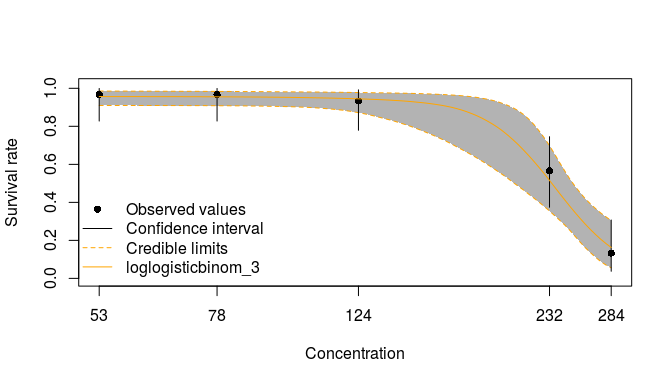Note that `survFitTT` will warn you if the estimated \(LC_{x}\) lie outside the range of tested concentrations, as in the following example:

``````data("cadmium1")
target.time = 21,
lcx = c(10, 20, 30, 40, 50))``````
``````## Warning: The LC50 estimation (model parameter e) lies outside the range of
## tested concentrations and may be unreliable as the prior distribution on
## this parameter is defined from this range !``````
``````plot(doubtful_fit, log.scale = TRUE, style = "ggplot", adddata = TRUE,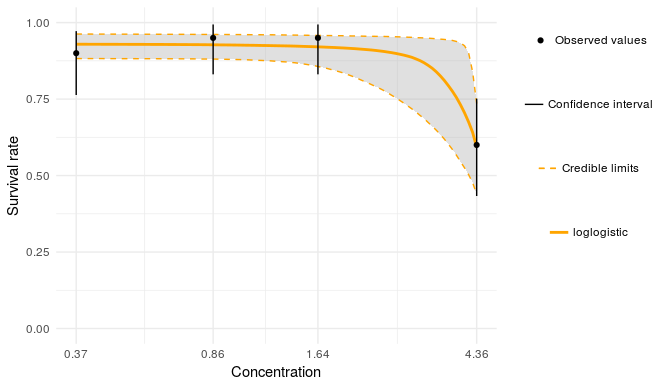In this example, the experimental design does not include sufficiently high concentrations, and we are missing measurements that would have a major influence on the final estimation. For this reason this result should be considered unreliable.

## Step 5: validate the model with a posterior predictive check

The fit can be further validated using so-called posterior predictive checks: the idea is to plot the observed values against the corresponding estimated predictions, along with their 95% credible interval. If the fit is correct, we expect to see 95% of the data inside the intervals.

``ppc(fit)``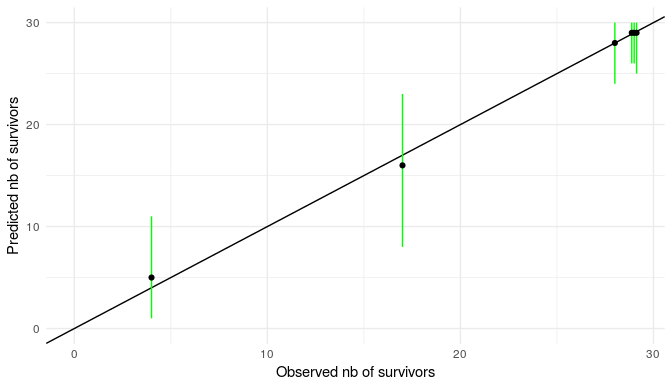In this plot, each black dot represents an observation made at a given concentration, and the corresponding number of survivors at target time is given by the value on the x-axis. Using the concentration and the fitted model, we can produce the corresponding prediction of the expected number of survivors at that concentration. This prediction is given by the y-axis. Ideally observations and predictions should coincide, so we’d expect to see the black dots on the points of coordinate \(Y = X\). Our model provides a tolerable variation around the predited mean value as an interval where we expect 95% of the dots to be in average. The intervals are represented in green if they overlap with the line \(Y=X\), and in red otherwise.

# Survival analysis using a toxico-kinetic toxico-dynamic (TK-TD) model

The steps for a TK-TD data analysis are absolutely analogous to what we described for the analysis at target time. Here the goal is to estimate the relationship between chemical compound concentration, time and survival rate.

## TK-TD model with constant exposure concentrations

Here is a typical session to analyse concentration-dependent time-course data using the so-called “Stochastic Death” (SD) model:

``````# (1) load dataset
data(propiconazole)

# (2) check structure and integrity of the dataset
survDataCheck(propiconazole)``````
``## No message``
``````# (3) create a `survData` object
dat <- survData(propiconazole)

# (4) represent the number of survivors as a function of time
plot(dat, pool.replicate = FALSE)``````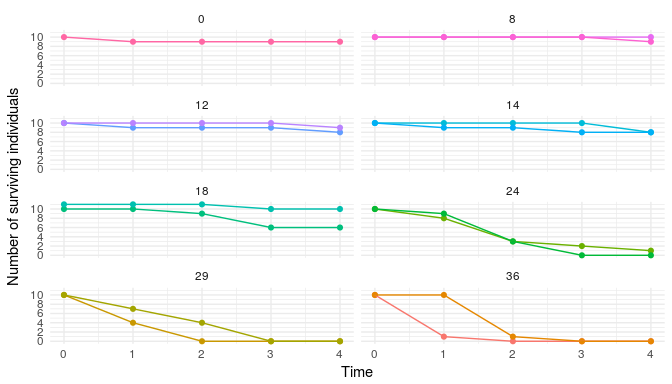``````# (5) check information on the experimental design
summary(dat)``````
``````##
## Number of replicates per time and concentration:
##     time
## conc 0 1 2 3 4
##   0  1 1 1 1 1
##   8  2 2 2 2 2
##   12 2 2 2 2 2
##   14 2 2 2 2 2
##   18 2 2 2 2 2
##   24 2 2 2 2 2
##   29 2 2 2 2 2
##   36 2 2 2 2 2
##
## Number of survivors (sum of replicates) per time and concentration:
##     0  1  2  3  4
## 0  10  9  9  9  9
## 8  20 20 20 20 19
## 12 20 19 19 19 17
## 14 20 19 19 18 16
## 18 21 21 20 16 16
## 24 20 17  6  2  1
## 29 20 11  4  0  0
## 36 20 11  1  0  0``````

### TK-TD model “SD”

To fit the Stochastic Death model, we have to specify the `model_type` as `"SD"`:

``````# (6) fit the TK-TD model SD
fit_cstSD <- survFit(dat, quiet = TRUE, model_type = "SD")``````

Then, the `summary` function provides parameters estimates as medians and 95% credible intervals.

``````# (7) summary of parameters estimates
summary(fit_cstSD)``````
``````## Summary:
##
## Priors of the parameters (quantiles) (select with '\$Qpriors'):
##
##  parameters    median      Q2.5     Q97.5
##          kd 4.157e-02 2.771e-04 6.236e+00
##          hb 1.317e-02 2.708e-04 6.403e-01
##           z 1.697e+01 8.121e+00 3.546e+01
##          kk 5.555e-03 1.016e-05 3.037e+00
##
## Posteriors of the parameters (quantiles) (select with '\$Qposteriors'):
##
##  parameters    median      Q2.5     Q97.5
##          kd 2.214e+00 1.614e+00 3.565e+00
##          hb 3.042e-02 1.454e-02 5.534e-02
##           z 1.706e+01 1.561e+01 1.883e+01
##          kk 1.247e-01 7.929e-02 1.906e-01``````

The `plot` function provides a representation of the fitting for each replicates

``plot(fit_cstSD)``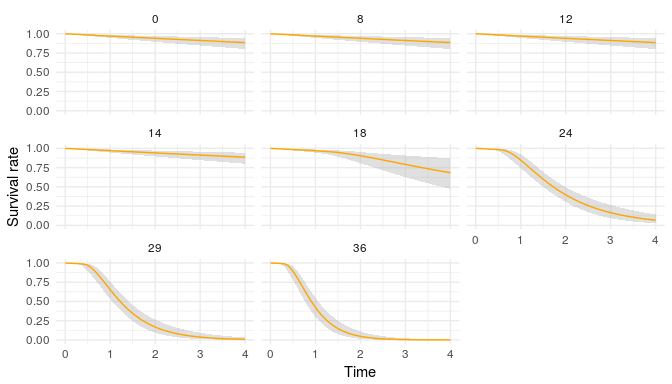Original data can be added by using the option `adddata = TRUE`

``plot(fit_cstSD, adddata = TRUE)``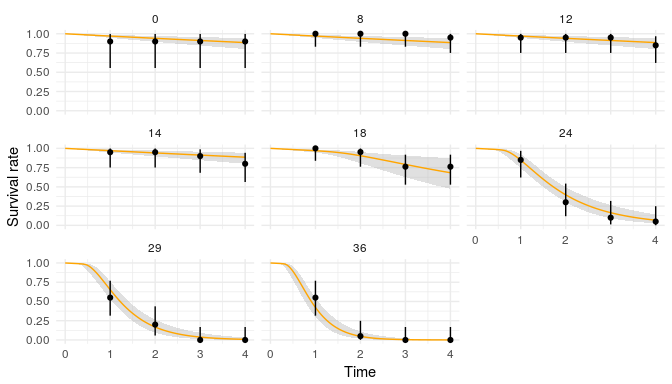A posterior predictive check is also possible using function `ppc`:

``ppc(fit_cstSD)``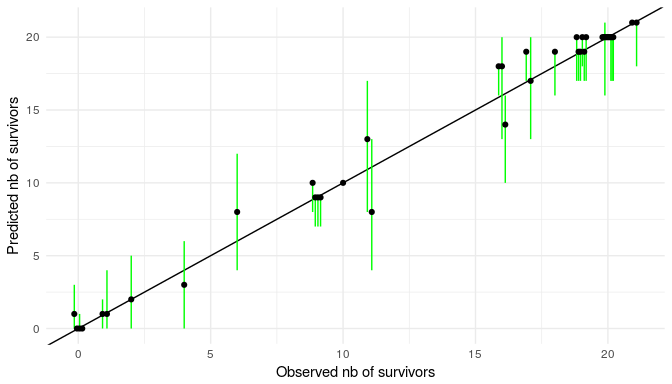Compared to the target time analysis, TK-TD modelling allows to compute and plot the lethal concentration for any x percentage and at any time-point. The chosen time-point can be specified with `time_LCx`, by default the maximal time-point in the dataset is used.

``````# LC50 at the maximum time-point:
LCx_cstSD <- LCx(fit_cstSD, X = 50)
plot(LCx_cstSD)``````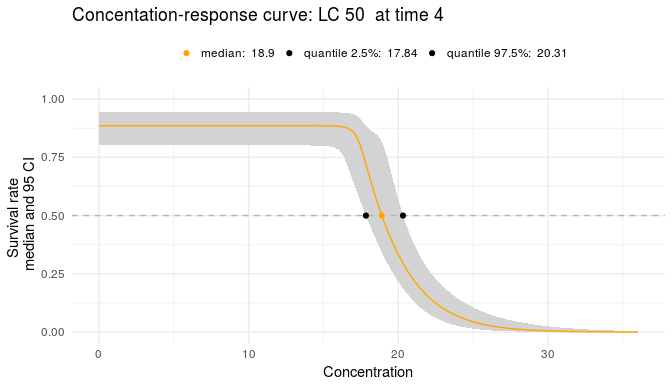``````# LC50 at time = 2
LCx(fit_cstSD, X = 50, time_LCx = 2) %>% plot()``````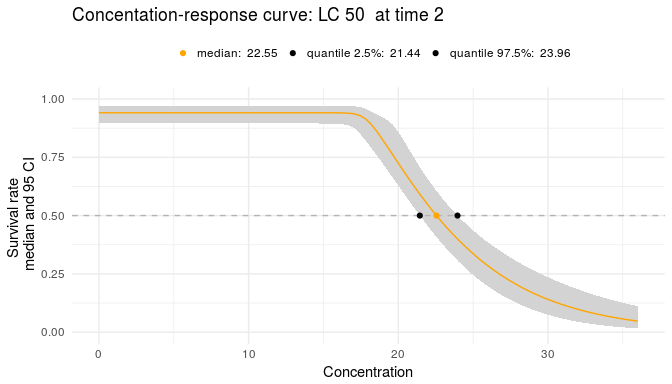``````## Note the use of the pipe operator, `%>%`, which is a powerful tool for clearly expressing a sequence of multiple operations.

Warning messages are returned when the range of concentrations is not appropriated for one or more LCx calculation(s).

``````# LC50 at time = 15
LCx(fit_cstSD, X = 50, time_LCx = 15) %>% plot()``````
``````## Warning in pointsLCx(df_dose, X_prop): No 95%inf for LC 50 in the range of
## concentrations under consideration: [ 0 ; 36 ]``````
``## Warning: Removed 1 rows containing missing values (geom_point).``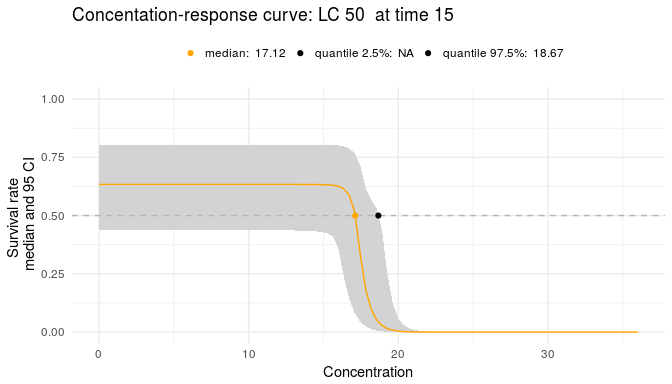### TK-TD model “IT”

The Individual Tolerance (IT) model is a variant of TK-TD survival analysis. It can also be used with `morse` as demonstrated hereafter. For the IT model, we have to specify the `model_type` as `"IT"`:

``fit_cstIT <- survFit(dat, quiet = TRUE, model_type = "IT")``

We can first get a summary of the estimated parameters:

``summary(fit_cstIT)``
``````## Summary:
##
## Priors of the parameters (quantiles) (select with '\$Qpriors'):
##
##  parameters    median      Q2.5     Q97.5
##          kd 4.157e-02 2.771e-04 6.236e+00
##          hb 1.317e-02 2.708e-04 6.403e-01
##       alpha 1.697e+01 8.121e+00 3.546e+01
##        beta 1.000e+00 1.259e-02 7.943e+01
##
## Posteriors of the parameters (quantiles) (select with '\$Qposteriors'):
##
##  parameters    median      Q2.5     Q97.5
##          kd 7.358e-01 5.408e-01 9.565e-01
##          hb 1.919e-02 4.672e-03 4.370e-02
##       alpha 1.798e+01 1.532e+01 2.050e+01
##        beta 6.858e+00 4.991e+00 9.268e+00``````
``````# OR
fit_cstIT\$estim.par``````
``````##   parameters      median         Q2.5       Q97.5
## 1         kd  0.73583144  0.540842905  0.95651945
## 2         hb  0.01918826  0.004672079  0.04369839
## 3      alpha 17.97678277 15.321918722 20.50171668
## 4       beta  6.85759549  4.991038961  9.26827860``````
``plot(fit_cstIT, adddata= TRUE)``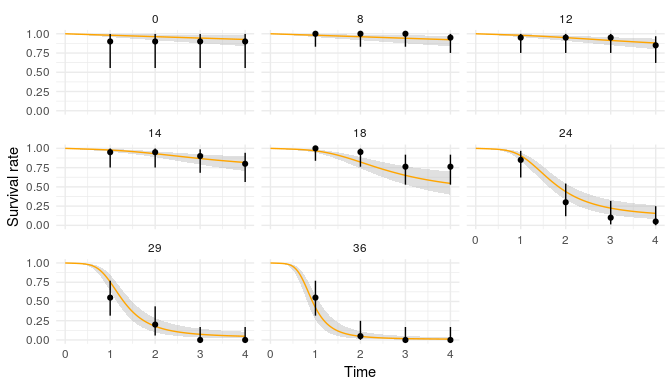``ppc(fit_cstIT)``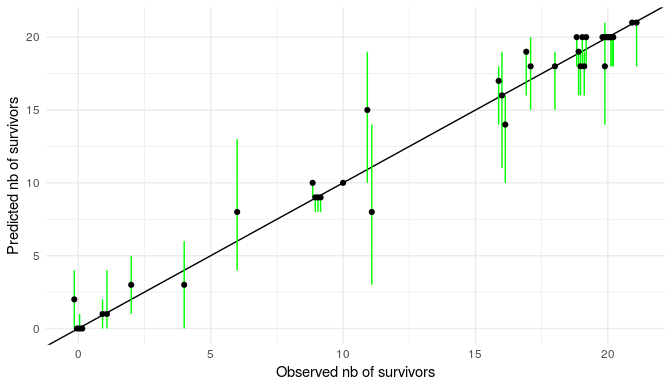``````# LC50 at the maximum time-point:
LCx_cstIT <- LCx(fit_cstIT, X = 50)
plot(LCx_cstIT)``````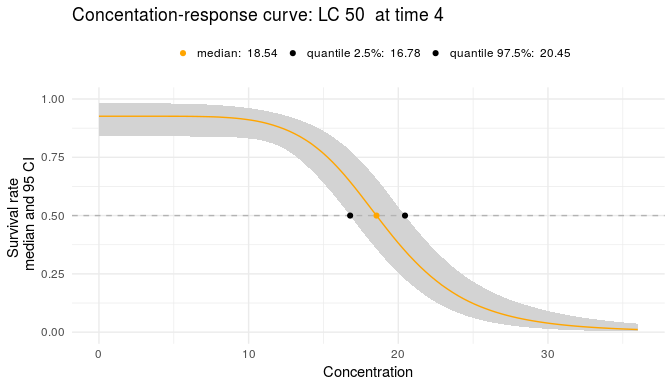``````# LC50 at time = 2
LCx(fit_cstIT, X = 50, time_LCx = 2) %>% plot()``````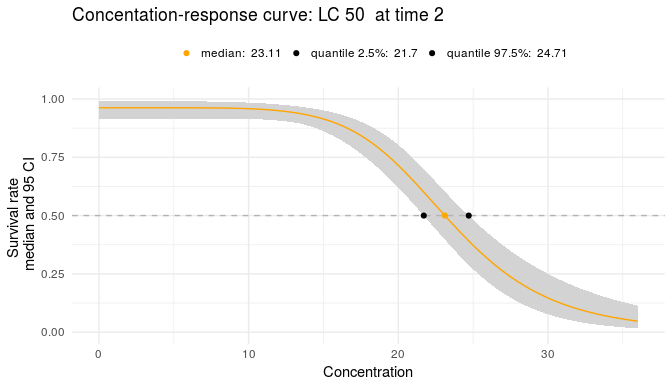``````# LC50 at time = 15
LCx(fit_cstIT, X = 50, time_LCx = 15) %>% plot()``````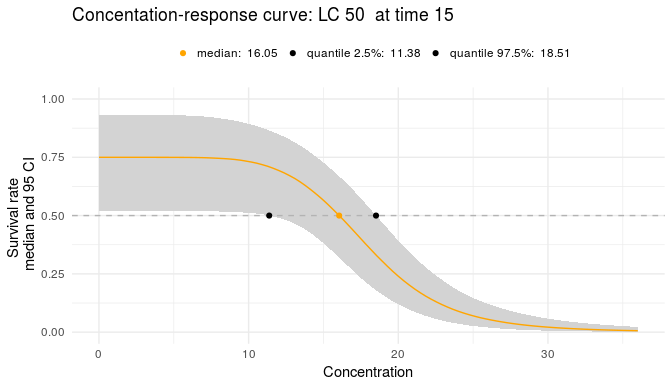## TK-TD model under time-variable exposure concentration

Here is a typical session fitting an SD or an IT model for a dataset under time-variable exposure scenario.

``````# (1) load dataset
data("propiconazole_pulse_exposure")

# (2) check structure and integrity of the dataset
survDataCheck(propiconazole_pulse_exposure)``````
``## No message``
``````# (3) create a `survData` object
dat <- survData(propiconazole_pulse_exposure)

# (4) represent the number of survivor as a function of time
plot(dat)``````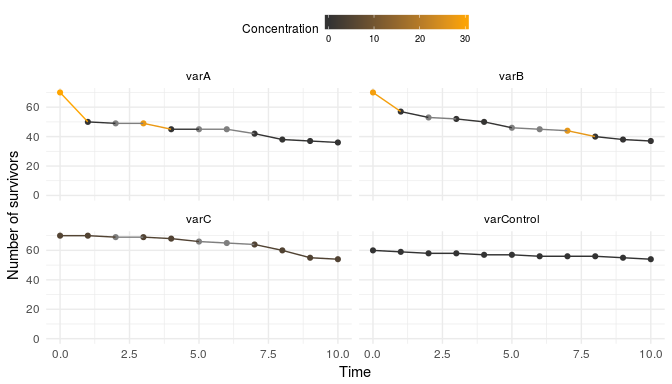``````# (5) check information on the experimental design
summary(dat)``````
``````##
## Occurence of 'replicate' for each 'time':
##             time
## replicate    0 0.96 1 1.96 2 2.96 3 3.96 4 4.96 4.97 5 5.96 6 6.96 7 7.96
##   varA       1    1 1    1 1    1 1    1 1    1    1 1    1 1    1 1    1
##   varB       1    1 1    1 1    1 1    1 1    1    1 1    1 1    1 1    1
##   varC       1    1 1    1 1    1 1    1 1    1    1 1    1 1    1 1    1
##   varControl 1    0 1    0 1    0 1    0 1    0    0 1    0 1    0 1    0
##             time
## replicate    8 9 9.96 10
##   varA       1 1    1  1
##   varB       1 1    1  1
##   varC       1 1    1  1
##   varControl 1 1    0  1
##
## Number of survivors per 'time' and 'replicate':
##             0 0.96  1 1.96  2 2.96  3 3.96  4 4.96 4.97  5 5.96  6 6.96  7
## varA       70   NA 50   NA 49   NA 49   NA 45   NA   NA 45   NA 45   NA 42
## varB       70   NA 57   NA 53   NA 52   NA 50   NA   NA 46   NA 45   NA 44
## varC       70   NA 70   NA 69   NA 69   NA 68   NA   NA 66   NA 65   NA 64
## varControl 60   NA 59   NA 58   NA 58   NA 57   NA   NA 57   NA 56   NA 56
##            7.96  8  9 9.96 10
## varA         NA 38 37   NA 36
## varB         NA 40 38   NA 37
## varC         NA 60 55   NA 54
## varControl   NA 56 55   NA 54
##
## Concentrations per 'time' and 'replicate':
##                0  0.96    1 1.96  2 2.96     3  3.96    4 4.96 4.97  5
## varA       30.56 27.93 0.00 0.26 NA 0.21 27.69 26.49 0.00 0.18 0.18 NA
## varB       28.98 27.66 0.00 0.27 NA 0.26  0.26  0.26 0.26 0.25 0.25 NA
## varC        4.93  4.69 4.69 4.58 NA 4.58  4.58  4.54 4.54 4.58 4.71 NA
## varControl  0.00    NA 0.00   NA  0   NA  0.00    NA 0.00   NA   NA  0
##            5.96  6 6.96     7  7.96    8    9 9.96   10
## varA       0.18 NA 0.14  0.14  0.18 0.18 0.00 0.00 0.00
## varB       0.03 NA 0.00 26.98 26.28 0.00 0.12 0.12 0.12
## varC       4.71 NA 4.60  4.60  4.59 4.59 4.46 4.51 4.51
## varControl   NA  0   NA  0.00    NA 0.00 0.00   NA 0.00``````

### TK-TD model “SD”

``````# (6) fit the TK-TD model SD
fit_varSD <- survFit(dat, quiet = TRUE, model_type = "SD")``````
``````## Warning: The estimation of the dominant rate constant (model parameter kd) lies
##     outside the range used to define its prior distribution which indicates that this
##     rate is very high and difficult to estimate from this experiment !``````
``````## Warning: The estimation of Non Effect Concentration threshold (NEC)
##       (model parameter z) lies outside the range of tested concentration
##       and may be unreliable as the prior distribution on this parameter is
##       defined from this range !``````
``````# (7) summary of the fit object
summary(fit_varSD)``````
``````## Summary:
##
## Priors of the parameters (quantiles) (select with '\$Qpriors'):
##
##  parameters    median      Q2.5     Q97.5
##          kd 2.683e-02 1.119e-04 6.434e+00
##          hb 8.499e-03 1.094e-04 6.606e-01
##           z 9.154e-01 3.212e-02 2.608e+01
##          kk 5.080e-02 4.342e-06 5.942e+02
##
## Posteriors of the parameters (quantiles) (select with '\$Qposteriors'):
##
##  parameters    median      Q2.5     Q97.5
##          kd 3.823e+00 1.604e+00 3.219e+01
##          hb 2.309e-02 1.613e-02 3.115e-02
##           z 2.097e+01 1.820e+00 3.539e+01
##          kk 7.935e-02 5.109e-03 7.046e-01``````
``plot(fit_varSD)``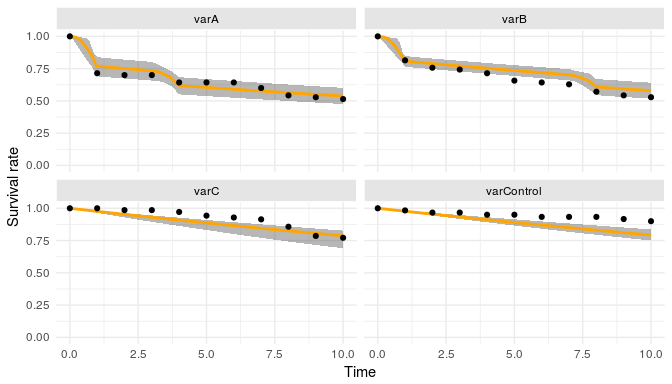``ppc(fit_varSD)``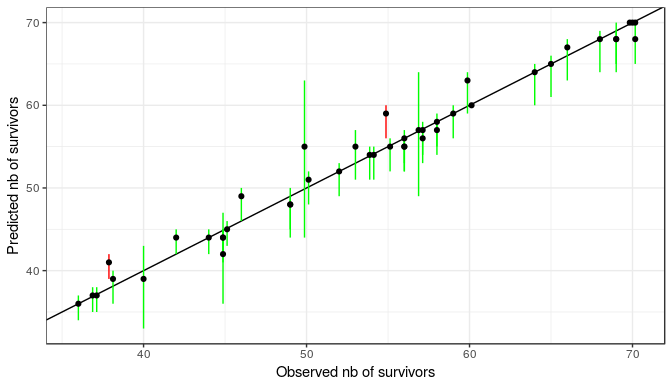``````# LC50 at time = 4
LCx_varSD <- LCx(fit_varSD, X = 50, time_LCx = 4, conc_range = c(0,100))
plot(LCx_varSD)``````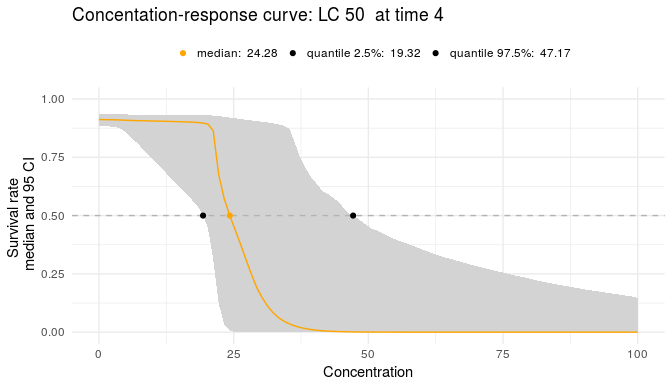``````# LC50 at time = 30
LCx(fit_varSD, X = 50, time_LCx = 30,  conc_range = c(0,100)) %>% plot()``````
``````## Warning in pointsLCx(df_dose, X_prop): No 95%inf for LC 50 in the range of
## concentrations under consideration: [ 0 ; 100 ]``````
``## Warning: Removed 1 rows containing missing values (geom_point).``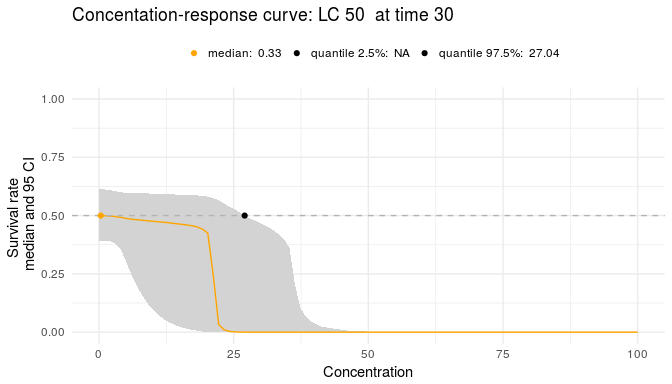### TK-TD model “IT”

``````# fit a TK-TD model IT
fit_varIT <- survFit(dat, quiet = TRUE, model_type = "IT")``````
``````## Warning: The estimation of the dominant rate constant (model parameter kd) lies
##     outside the range used to define its prior distribution which indicates that this
##     rate is very high and difficult to estimate from this experiment !``````
``````## Warning: The estimation of log-logistic median (model parameter alpha)
##       lies outside the range of tested concentration and may be unreliable as
##       the prior distribution on this parameter is defined from this range !``````
``````# (7) summary of the fit object
summary(fit_varIT)``````
``````## Summary:
##
## Priors of the parameters (quantiles) (select with '\$Qpriors'):
##
##  parameters    median      Q2.5     Q97.5
##          kd 2.683e-02 1.119e-04 6.434e+00
##          hb 8.499e-03 1.094e-04 6.606e-01
##       alpha 9.154e-01 3.212e-02 2.608e+01
##        beta 1.000e+00 1.259e-02 7.943e+01
##
## Posteriors of the parameters (quantiles) (select with '\$Qposteriors'):
##
##  parameters    median      Q2.5     Q97.5
##          kd 3.790e-01 5.562e-04 1.680e+01
##          hb 2.413e-02 1.604e-02 3.296e-02
##       alpha 1.733e+01 2.021e-01 3.773e+01
##        beta 2.152e+00 4.776e-01 2.158e+01``````
``plot(fit_varIT)``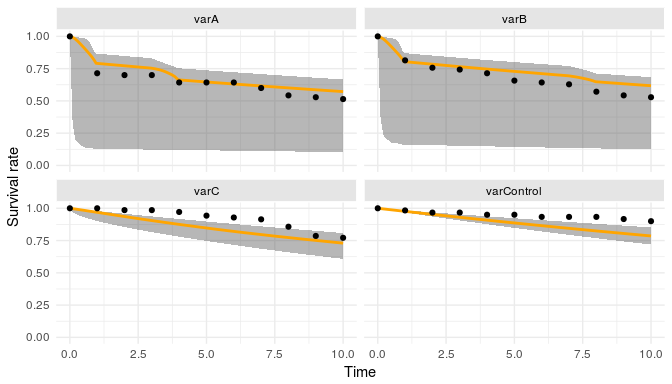``ppc(fit_varIT)``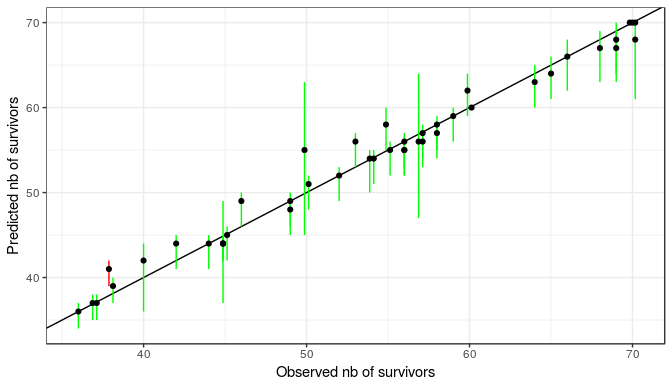``````# LC50 at time = 4
LCx(fit_varIT, X = 50, time_LCx = 4, conc_range = c(0,200)) %>% plot()``````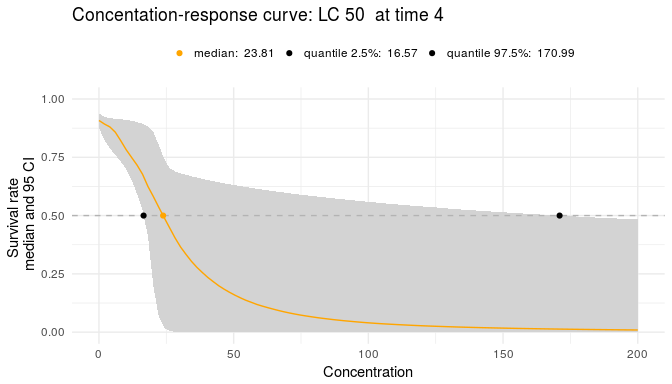``````# LC50 at time = 30
LCx(fit_varIT, X = 50, time_LCx = 30, conc_range = c(0,100)) %>% plot()``````
``````## Warning in pointsLCx(df_dose, X_prop): No median for LC 50 in the range of
## concentrations under consideration: [ 0 ; 100 ]``````
``````## Warning in pointsLCx(df_dose, X_prop): No 95%inf for LC 50 in the range of
## concentrations under consideration: [ 0 ; 100 ]``````
``## Warning: Removed 2 rows containing missing values (geom_point).``